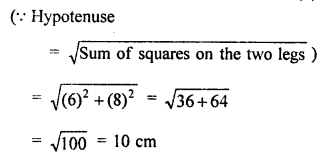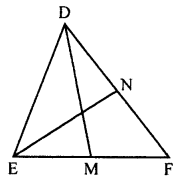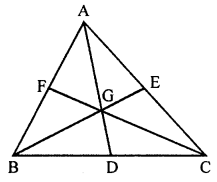## ML Aggarwal Class 7 Solutions for ICSE Maths Chapter 11 Triangles and its Properties Objective Type Questions

Mental Maths

Question 1.
Fill in the blanks:
(i) A triangle has at least ………… acute angles.
(ii) A triangle cannot have more than ……… right angle.
(iii) A triangle cannot have more than ………… obtuse angle.
(iv) In every triangle, the sum of (interior) angles of a triangle = ……….. right angles.
(v) In every triangle, an exterior angle + adjacent interior angle = ………… degrees.
(vi) In every triangle, an exterior angle = sum of the …….. interior opposite angles.
(vii) In a right-angled triangle, if one of the acute angles measures 25° then the measure of the other acute angle is …………
Solution:
(i) A triangle has at least two acute angles.
(ii) A triangle cannot have more than one right angle.
(iii) A triangle cannot have more than one obtuse angle.
(iv) In every triangle, the sum of (interior) angles of a triangle = two right angles.
(v) In every triangle, an exterior angle + adjacent interior angle = 180° degrees.
(vi) In every triangle, an exterior angle = sum of the two interior opposite angles.
(vii) In a right-angled triangle, if one of the acute angles measures 25°
then the measure of the other acute angle is 65°.

Question 2.
State whether the following statements are true (T) or false (F):
(i) A triangle can have all three angles with a measure greater than 60°.
(ii) A triangle can have all three angles with a measure of less than 60°.
(iii) If an exterior angle of a triangle is a right angle, then each of its interior opposite angles is acute.
(iv) In a right-angled triangle, the sum of two acute angles is 90°.
(v) If all the three sides of a triangle are equal, then it is called a scalene triangle.
(vi) Every equilateral triangle is an isosceles triangle.
(vii) Every isosceles triangle must be an equilateral triangle.
(viii) Each acute angle of an isosceles right-angled triangle measures 60°.
(ix) A median of a triangle always lies inside the triangle.
(x) An altitude of a triangle always lies outside the triangle.
(xii) In a triangle, the sum of squares of two sides is equal to the square of the third side.
Solution:
(i) A triangle can have all three angles with a measure greater than 60°. (False)
Correct:
The sum of three angles = 180°
(ii) A triangle can have all three angles with a measure of less than 60°. (False)
Correct:
The sum of three angles = 180°
(iii) If an exterior angle of a triangle is a right angle,
then each of its interior opposite angles is acute. (True)
(iv) In a right-angled triangle, the sum of two acute angles is 90°. (True)
(v) If all the three sides of a triangle are equal,
then it is called a scalene triangle. (False)
Correct:
It is an equilateral triangle.
(vi) Every equilateral triangle is an isosceles triangle. (True)
(vii) Every isosceles triangle must be an equilateral triangle. (False)
Correct:
An isosceles triangle has any two sides equal
but an equilateral triangle has three sides equal.
(viii) Each acute angle of an isosceles right-angled triangle measures 60°. (False)
Correct:
Each acute angle will be of 45°, not 60°.
(ix) A median of a triangle always lies inside the triangle. (True)
(x) An altitude of a triangle always lies outside the triangle. (False)
Correct:
Not always, but in some cases only.
(xii) In a triangle, the sum of squares of two sides is
equal to the square of the third side. (False)
Correct:
Only in right triangle, the sum of squares on two sides is
equal to the square on the hypotenuse.

Multiple Choice Questions

Choose the correct answer from the given four options (3 to 17):
Question 3.
A triangle formed by the sides of lengths 4.5 cm, 6 cm, and 4.5 cm is
(a) scalene
(b) isosceles
(c) equilateral
(d) none of these
Solution:
A triangle formed by the sides of lengths
4.5 cm, 6 cm, and 4.5 cm is isosceles. (b)

Question 4.
The number of medians in a triangle is
(a) 1
(b) 2
(c) 3
(d) 4
Solution:
The number of medians in a triangle is 3. (c)

Question 5.
An exterior angle of a triangle is 125°. If one of the two interior opposite angles is 55° then the other interior opposite angle is
(a) 70°
(b) 55°
(c) 60°
(d) 80°
Solution:
An exterior angle of a triangle is 125°.
If one of the two interior opposite angles is 55°
then the other interior opposite angle is 125° – 55° = 70° (a)

Question 6.
In a ∆ABC, if ∠A = 40° and ∠B = 55° then ∠C is
(a) 75°
(b) 80°
(c) 95°
(d) 85°
Solution:
In a ∆ABC, if ∠A = 40° and ∠B = 55°
then ∠C is 180° – (40° + 55°) = 180° – 95° = 85° (d)

Question 7.
If the angles of a triangle are 35°, 35°, and 110°, then it is
(a) an isosceles triangle
(b) an equilateral triangle
(c) a scalene triangle
(d) right-angled triangle
Solution:
If the angles of a triangle are 35°, 35°, and 110°,
then it is an isosceles triangle. (a)

Question 8.
A triangle whose two angles measure 30° and 120° is
(a) scale
(b) isosceles
(c) equilateral
(d) none of these
Solution:
A triangle whose two angles measure
30° and 120° is an isosceles triangle. (b)

Question 9.
A triangle can have two
(a) right angles
(b) obtuse angles
(c) acute angles
(d) straight angles
Solution:
A triangle can have two acute angles. (c)

Question 10.
A triangle whose angles measure 35°, 35° and 90° is
(a) acute angled
(b) right-angled
(c) obtuse-angled
(d) isosceles
Solution:
A triangle whose angles measure
35°, 35° and 90° is right-angled. (b)

Question 11.
A triangle is not possible whose angles measure
(a) 40°, 65°, 75°
(b) 50°, 56°, 74°
(c) 72°, 63°, 45°
(d) 67°, 42°, 81°
Solution:
A triangle is not possible whose angles measure 67°, 42°, 81°. (d)
(Sum is more than 180°)

Question 12.
If in an isosceles triangle, each of the base angles is 40°, then the triangle is
(a) right-angled triangle
(b) acute-angled triangle
(c) obtuse-angled triangle
(d) isosceles right-angled triangle
Solution:
If in an isosceles triangle, each of the base angles is 40°,
then the triangle is an obtuse angled triangle. (c)

Question 13.
A triangle is not possible with sides of lengths (in cm)
(a) 6, 4, 10
(b) 5, 3, 7
(c) 7, 8, 9
(d) 3.6, 5.4, 8
Solution:
A triangle is not possible with sides of lengths (in cm) 6, 4, 10. (a)
(Sum of two sides must be greater than its third side)

Question 14.
Which of the following can be the length of the third side of a triangle whose two sides measure 18 cm and 14 cm?
(a) 32 cm
(b) 3 cm
(c) 4 cm
(d) 5 cm
Solution:
The length of the third side of a triangle
whose two sides measure 18 cm and 14 cm = 5 cm (d)
(∵ The sum of any two sides must be greater than its third side)

Question 15.
In a right-angled triangle, the lengths of two leg’s are 6 cm and 8 cm. The length of the hypotenuse is
(a) 14 cm
(b) 10 cm
(c) 11 cm
(d) 12 cm
Solution:
In a right-angled triangle,
the lengths of two legs are 6 cm and 8 cm.
The length of the hypotenuse is 10 cm. (b)Question 16.
If the dimensions of a rectangle are 15 m and 8 m, then the length of a diagonal is
(a) 7 m
(b) 23 m
(c) 17 m
(d) 20 m
Solution:
If the dimensions of a rectangle are 15 m and 8 m,
then the length of a diagonal is 17 m.
(∵ 172 = 82 + 152) (c)

Question 17.
If p, q, and r are the lengths of the three sides of a triangle, then which of the following statements is correct?
(a) p + q = r
(b) p + q < r (c) p + q > r
(d) p – q > r
Solution:
p + q > r (c)
(∵ Sum of any two sides of a triangle is greater than its third side)

Higher Order Thinking Skills (HOTS)

Question 1.
In ∆DEF, DM and EN are two medians. Prove that 3(DF + EF) > 2(DM + EN).
Solution:
In ∆DEF, DM and EN are two medians.To prove : 3(DF + EF) > 2(DM + EN)
Proof: In ∆DMF
DF + MF > DM
(Sum of any two sides is greater than its third side)
⇒ DF = $$\frac { 1 }{ 2 }$$ EF > DM ……(i)
(∵ M is mid-point of EF)
Similarly in ∆EFN,
EF + NF > EN
⇒ EF + $$\frac { 1 }{ 2 }$$ DF > EN …….(ii)
(FD + $$\frac { 1 }{ 2 }$$ EF + EF + $$\frac { 1 }{ 2 }$$ DF) > (DM + EN)
⇒ ($$\frac { 3 }{ 2 }$$ FD + $$\frac { 3 }{ 2 }$$ EF) > (DM + EN)
⇒ $$\frac { 3 }{ 2 }$$ (FD + EF) > (DM) + EN)
⇒ 3(FD + EF) > 2(DM + EN)

Question 2.
In a ∆ABC, medians AD, BE and CF intersect each other at point G. Prove that 3(AB + BC + CA) > 2(AD + BE + CF).
Solution:
In ∆ABC, medians AD, BE and CF intersect each other at G.To prove :
3(AB + BC + CA) > 2(AD + BE + CF)
Proof: In ∆ABC, D, E and F are the midpoints of
BC, CA and AB respectively
In ∆ABD,
Sum of any two sides is greater than its third side
⇒ AB + $$\frac { 1 }{ 2 }$$ RC > AD ……(i)
Similarly in ∆BCE
BC + CE > BE
⇒ BC + $$\frac { 1 }{ 2 }$$ AC > BE ……(ii)
and in ∆CAF,
CA + AF > CF
⇒ CA + $$\frac { 1 }{ 2 }$$ AB > CF ……(iii)
AB + $$\frac { 1 }{ 2 }$$ BC + BC + $$\frac { 1 }{ 2 }$$ AC + AC + $$\frac { 1 }{ 2 }$$ AB > AD + BE + CF
⇒ $$\frac { 3 }{ 2 }$$ AB + $$\frac { 3 }{ 2 }$$ BC + $$\frac { 3 }{ 2 }$$ CA > AD + BE + CF
⇒ $$\frac { 3 }{ 2 }$$ (AB + BC + CA) > (AD + BE + CF)